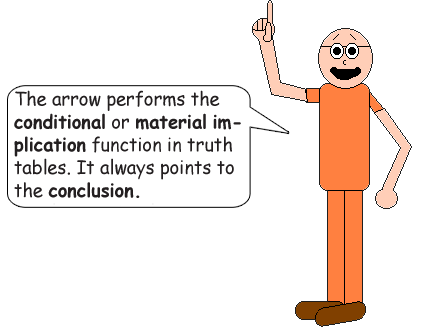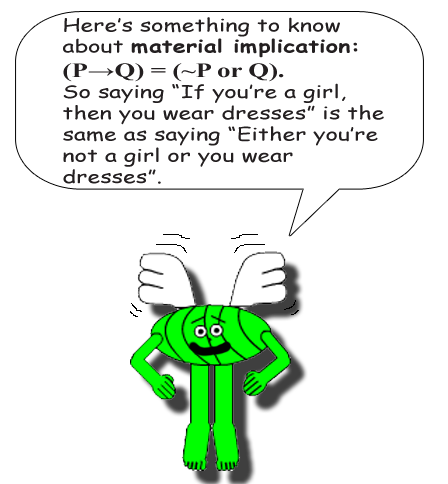# The Staircase of Falsity

## Here's another math trick that's about logic instead of numbers! Can you guess how this math trick got its name?

 P Q ~P ~Q P→~Q P→Q ~P→~Q ~P→Q P←~Q P←Q ~P←~Q ~P←Q TRUE TRUE FALSE FALSE FALSE TRUE TRUE TRUE TRUE TRUE TRUE FALSE TRUE FALSE FALSE TRUE TRUE FALSE TRUE TRUE TRUE TRUE FALSE TRUE FALSE TRUE TRUE FALSE TRUE TRUE FALSE TRUE TRUE FALSE TRUE TRUE FALSE FALSE TRUE TRUE TRUE TRUE TRUE FALSE FALSE TRUE TRUE TRUE

Look at the truth values of the orange columns. Now look at the truth values of the blue columns. They form a staircase of falsity as you move to the next column! The orange columns go downward & the blue ones go upward!Conditional statements are only false when the input behind the arrow is true but the input in front of the arrow is false.

Some of the compound statements in the truth table above are logically equivalent! Can you guess which ones? I'll give you 1 example: (~P→~Q) = (P←Q)!